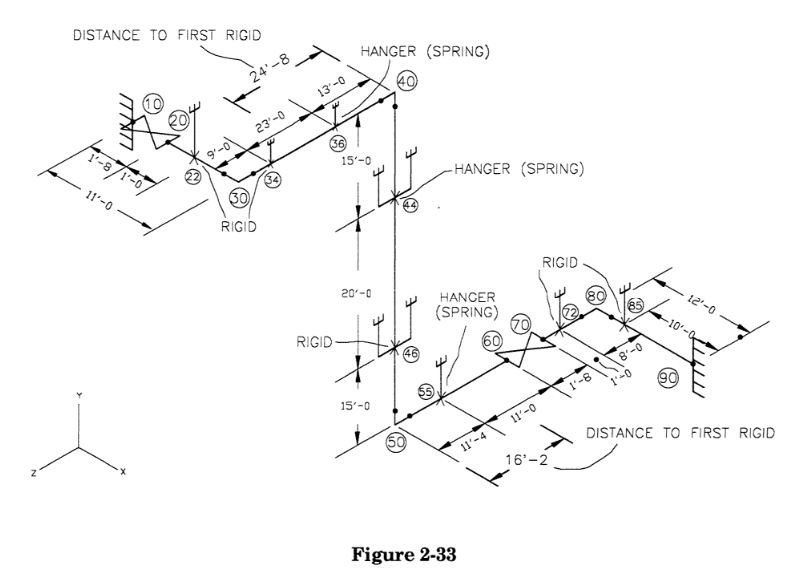Search

# 2.4.5 Restraint Placement Using Distance to First Rigid Criteria

If the above procedure is followed exactly, it is likely that almost all support locations will show some vertical movement, and will therefore require springs. For economic reasons, it is best to try to limit the number of springs by using rigid supports at locations with small movements.

How can this be done? The analyst can potentially impose zero movement at points where it is safe from an expansion stress point of view; if there is no vertical pipe run between points of zero growth, all supports along that run should have zero growth as well. For example:The question is where can rigid restraints be placed without causing expansion problems? If the expansion displacement is known at a given point, the minimum distance to the first rigid restraint can be calculated using the guided cantilever stress formula:This can be illustrated by an example. In the system depicted in Figure 2-12, there are eight hanger locations. If the procedure described in Section 2.4.4 is followed to the letter, there will certainly be thermal growth at all of these locations, so eight springs will be selected. In order to reduce this number, some engineers impose a rigid displacement criteria — for example, if the displacement at the hanger location is less than some value (such as 0.1 inches), they will select a rigid rod rather than a spring. (Note that this procedure is not fool-proof, since using rigid rods at locations such as the tops of risers or near equipment nozzles may cause lock-up or lift-off despite having "free-thermal" displacements of less than 0.1 inches; therefore application of a procedure such as this must be reviewed carefully.) The reader can confirm that re-running this problem with a rigid displacement criteria of 0.1 inches actually leads to a reduction in springs used, with only five selected.

The engineer can do better than that by pre-selecting potential hanger candidates through the use of the distance-to-first-rigid criteria. All of the vertical thermal growth in this problem is generated by the riser between node points 40 and 50; we can calculate that growth as:

Δ = 50 x 12 x 1.88 E-3 = 1.13 in

The engineer can direct part of this growth upward, to be absorbed by the horizontal portion between node points 10 and 40, and the rest of it downward to be absorbed by the horizontal portion between node points 50 and 90, by requiring a rigid restraint at one of the hanger locations on the riser. Which of these two hangers is a better candidate for a rigid support? Since the expansion stress is inversely proportional to the square of the lengths of the resisting legs, it is logical to direct the greater part of the thermal growth upward, since a quick check reveals that the spans from node point 10 to 40 are longer than the spans between node points 50 and 90. Therefore, the hanger at node point 46 should be selected as the rigid support, and the other one (at node point 44) must be a spring. This will force a thermal displacement of (35 / 50) x 1.13 = 0.79 inches upward, and a displacement of (15 / 50) x 1.13 = 0.34 inches downward.

Looking at the portion of the system from node point 10 to 40, one first considers the hanger located at node point 36. A rigid support may be placed here if the span from node point 36 to 40 is long enough to absorb the displacement of 0.79 inches, or, if the distance from node point 36 to 40 is greater than the calculated distance to the first rigid, which is (using Sall = 10,000 psi):The distance between node points 36 and 40 is only 13 feet, which exceeds the calculated distance to the first rigid, so the support at node point 36 must be a spring. Looking at the next support point, at node point 34, the distance from the riser is 36 feet, exceeding the allowable distance to the first rigid. This indicates that a rigid support maybe placed at node point 34 without causing undue expansion problems. Next, since the pipe is being restrained vertically at node point 34, there are no imposed displacements at the nozzle at node point 10, and there are no risers between node points 10 and 34, all locations between the nozzle and the restraint will also have no vertical thermal growth. This means that a rigid support, rather than a spring, may be used at node point 22 as well.

The lower part of the piping system must absorb only 0.34 inches. The minimum distance to the first rigid required to absorb this displacement is:The distance between the riser and the hanger location at node point 55 is ll'-4, which is within the minimum distance to the first rigid. Therefore, the support at this location should be a spring. The distance from the riser to the support at node point 72 is 25 feet, which exceeds the minimum distance criteria, and may therefore be a rigid support. This rigid support holds the pipe to a zero vertical displacement, which when considered with the anchor at node point 90, and the lack of risers in-between, means that the hanger at node point 85 may also be a rigid support.

By pre-declaring these supports to be rigid supports, the engineer limits the number of possible spring hanger candidates in this case to a maximum of three, a savings on hardware beyond that achieved though use of the rigid rod displacement criteria method. It should be noted that declaring that a support to be a rigid support does not mean that it must be constructed as a two-way restraint; if there is no net uplift force, it may be built as a simple rod hanger.